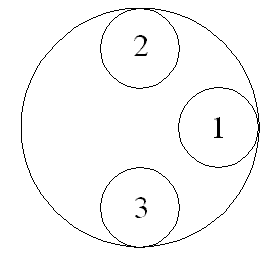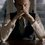# A Missouri State Archive problem

This is the problem link. How about we discuss this problem here?

I will just put it here -

Two children play a game with three coins each having a radius of 1 unit. Each turn of the game consists of sliding one coin between the other two (without moving either of them). A given coin may not be moved in two consecutive turns. What is the smallest circular region in which it is possible for the game to be played indefinitely? For example, in the circular region shown below, coin 1 can be slid between coins 2 and 3, but then neither coin 2 nor coin 3 can then be slid between the other two.Note by Kartik Sharma
4 years, 5 months ago

This discussion board is a place to discuss our Daily Challenges and the math and science related to those challenges. Explanations are more than just a solution — they should explain the steps and thinking strategies that you used to obtain the solution. Comments should further the discussion of math and science.

When posting on Brilliant:

• Use the emojis to react to an explanation, whether you're congratulating a job well done , or just really confused .
• Ask specific questions about the challenge or the steps in somebody's explanation. Well-posed questions can add a lot to the discussion, but posting "I don't understand!" doesn't help anyone.
• Try to contribute something new to the discussion, whether it is an extension, generalization or other idea related to the challenge.

MarkdownAppears as
*italics* or _italics_ italics
**bold** or __bold__ bold
- bulleted- list
• bulleted
• list
1. numbered2. list
1. numbered
2. list
Note: you must add a full line of space before and after lists for them to show up correctly
paragraph 1paragraph 2

paragraph 1

paragraph 2

[example link](https://brilliant.org)example link
> This is a quote
This is a quote
    # I indented these lines
# 4 spaces, and now they show
# up as a code block.

print "hello world"
# I indented these lines
# 4 spaces, and now they show
# up as a code block.

print "hello world"
MathAppears as
Remember to wrap math in $$ ... $$ or $ ... $ to ensure proper formatting.
2 \times 3 $2 \times 3$
2^{34} $2^{34}$
a_{i-1} $a_{i-1}$
\frac{2}{3} $\frac{2}{3}$
\sqrt{2} $\sqrt{2}$
\sum_{i=1}^3 $\sum_{i=1}^3$
\sin \theta $\sin \theta$
\boxed{123} $\boxed{123}$

Sort by:

Interesting question. What are your thoughts?

If we have an infinitely large circle, can the game continue indefinitely? If yes, can we bound the table in any way?

Staff - 4 years, 5 months ago

You can check my comment above.

- 4 years, 5 months ago

Well, it's not said where a coin can end up after a move, and if not necessarily back in contact with the circular court, then what does it mean to be "slid between the other two coins"?

Let's assume that the moved coin has to be in contact with the circular court. Then since that coin cannot be immediately moved again, then either of other coins is going to move such a way to bring all three coins closer, and this will continue until no further move can be made, regardless of the size of the circular court.

Maybe we need to relax the rules or terms of the game, or motivation of the players?

- 4 years, 5 months ago

Yes, exactly. My first was the same as yours. But maybe we are missing some point since it is an "established problem".

One possibility might be the following -

Let's say player A starts the game. Suppose initially coins $1,2,3$(in that order) are placed on the circumference such that they just touch each other. Now, "sliding between the other two coins" does not necessarily means "sliding exactly in between bisecting the space."

We will assume that prime objective of the players is not to win but to let the game continue indefinitely.

So, player A will move coin $2$ such that it may just touch coin $1$. Then player B moves coin $1$ to touch one of the other coins and so on.

I think that since we have freedom to move the coin to be slid anywhere, there is a possibility that this is indeed a very valid problem although I have thought much.

- 4 years, 5 months ago

I see that I am confused myself.

- 4 years, 5 months ago

The coin does not need to hit the edge of the court. It just need to pass (completely) through the line connecting the centers of the 2 circles.

You make a good observation here (but not yet phrased as a simple characteristic) for a condition under which the game must eventually end.

Staff - 4 years, 5 months ago

I'm gonna post a problem pretty soon based on this game.

- 4 years, 5 months ago

I've left out mention of this note because that "This" site could eventually post a solution in the future.

- 4 years, 5 months ago

That is one good problem here! Couldn't even think of a good approach for this problem! Nice problem, Kartik!

I hope that there is no solution posted, and if this happens, then the challenge is spoiled. XD Good luck for those who want to try out the difficult problem!

- 4 years, 4 months ago

3 units

- 4 years, 4 months ago

@chandan kumar,it cannot be 3 units, so dont post wrong answers

- 4 years, 4 months ago

@Kartik Sharma- the answer is 25pi/4 units because the distance bw 2 circles must be 5r for a coin to pass and if we take this as diameter is 5r so answer is equal to 25/4pi, now it is correct, bcuz I am just so good in maths, just try to Under

- 4 years, 4 months ago

As Kartik mentioned before, please don't post answer here. Show the solution.

- 4 years, 4 months ago

25pi units, I know I am right because I am always right, thnx

- 4 years, 4 months ago

Please refrain from adding such comments on a problem solving discussion. If you think it is $25\pi$, then add a solution not an answer.

- 4 years, 4 months ago